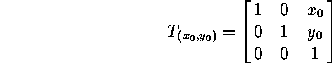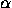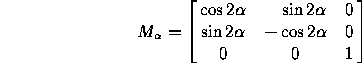# 2.2 Formulas for Symmetries in Homogeneous Coordinates

All isometries of the plane can be expressed in homogeneous coordinates in terms of multiplication by a matrix. This fact is useful in the implementation of these transformations on the computer, for example. It also means that the successive application of transformations reduces to matrix multiplication. The corresponding matrices are as follows:

Translation by (x,y):Rotation througharound the origin:Reflection in a line going through the origin and making an anglewith the x-axis:From this one can deduce all other transformations: for example, to find the matrix for a rotation througharound an arbitrary point P=(x,y), we apply a translation by -(x,y) to move P to the origin, then a rotation througharound the origin, then a translation by (x,y):(notice the order of the multiplication).

Next: 2.3 Formulas for Symmetries in Polar Coordinates
Up: 2 Plane Symmetries or Isometries
Previous: 2.1 Formulas for Symmetries in Cartesian CoordinatesThe Geometry Center Home Page

Silvio Levy
Wed Oct 4 16:41:25 PDT 1995

This document is excerpted from the 30th Edition of the CRC Standard Mathematical Tables and Formulas (CRC Press). Unauthorized duplication is forbidden.